# 8000字长文让你彻底了解 Java 8 的 Lambda、函数式接口、Stream 用法和原理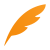Java 8 是在 2014 年发布的，实话说，风筝我也是在 Java 8 发布后很长一段时间才用的 Stream，因为 Java 8 发布的时候我还在 C# 的世界中挣扎，而使用 Lambda 表达式却很早了，因为 Python 中用 Lambda 很方便，没错，我写 Python 的时间要比 Java 的时间还长。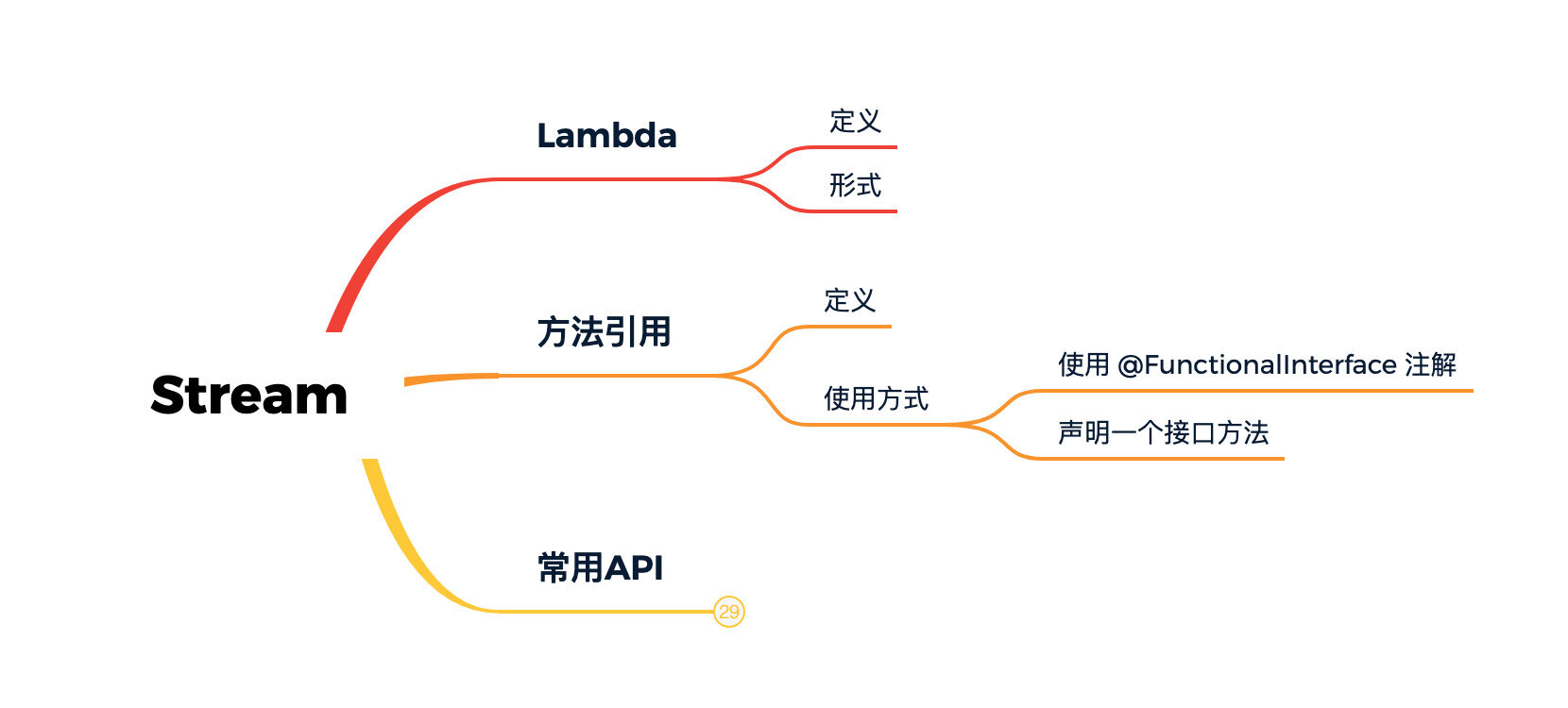## Lambda 表达式

Lambda 表达式是一个匿名函数，Lambda表达式基于数学中的[λ演算](https://baike.baidu.com/item/λ演算)得名，直接对应于其中的lambda抽象，是一个匿名函数，即没有函数名的函数。Lambda表达式可以表示闭包。

// 无参数，无返回值() -> log.info("Lambda") // 有参数，有返回值(int a, int b) -> { a+b }

log.info("Lambda");private int plus(int a, int b){  	return a+b;}

new Thread(new Runnable() {    @Override    public void run() {        System.out.println("快速新建并启动一个线程");    }}).run();

new Thread(()->{    System.out.println("快速新建并启动一个线程");}).run();

Lambda 表达式简化了匿名内部类的形式，可以达到同样的效果，但是 Lambda 要优雅的多。虽然最终达到的目的是一样的，但其实内部的实现原理却不相同。

## 方法引用

Function<String, Integer> s = Integer::parseInt;Integer i = s.apply("10");

Comparator<Integer> comparator = Integer::compare;int result = comparator.compare(100,10);

IntBinaryOperator intBinaryOperator = Integer::compare;int result = intBinaryOperator.applyAsInt(10,100);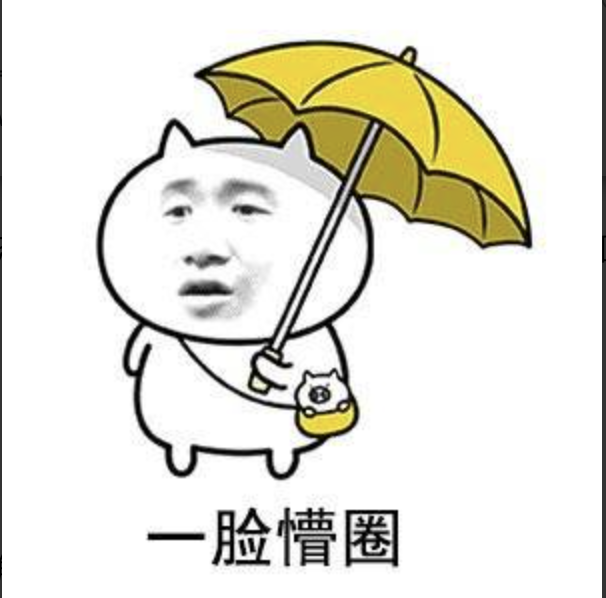Q：什么样的方法可以被引用？

A：这么说吧，任何你有办法访问到的方法都可以被引用。

Q：返回值到底是什么类型？

A：这就问到点儿上了，上面又是 Function、又是Comparator、又是 IntBinaryOperator的，看上去好像没有规律，其实不然。

@FunctionalInterfacepublic interface Function<T, R> {    R apply(T t);}

public static int parseInt(String s) throws NumberFormatException {    return parseInt(s,10);}

Integer.compare方法定义如下：

public static int compare(int x, int y) {    return (x < y) ? -1 : ((x == y) ? 0 : 1);}

@FunctionalInterfacepublic interface Comparator<T> {    int compare(T o1, T o2);}@FunctionalInterfacepublic interface IntBinaryOperator {    int applyAsInt(int left, int right);}

JDK 中定义了很多函数式接口，主要在 java.util.function包下，还有 java.util.Comparator 专门用作定制比较器。另外，前面说的 Runnable也是一个函数式接口。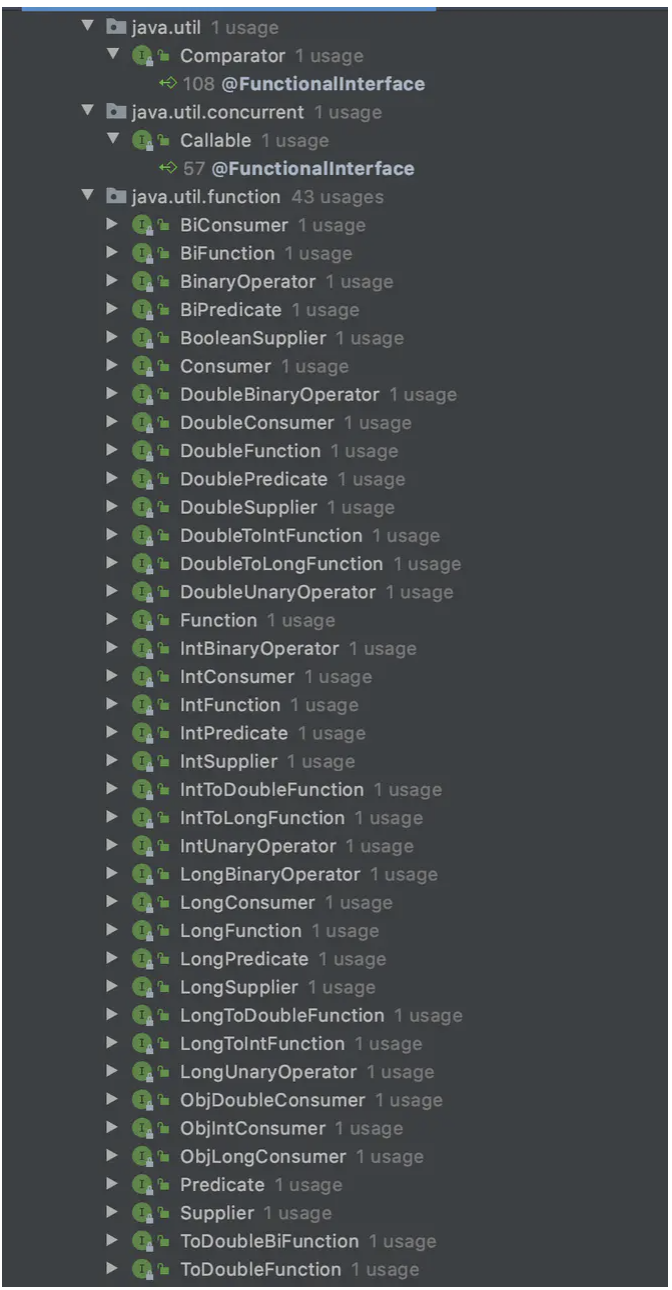## 自己动手实现一个例子

1. 定义一个函数式接口，并添加一个方法

@FunctionalInterfacepublic interface KiteFunction<T, R, S> {    /**     * 定义一个双参数的方法     * @param t     * @param s     * @return     */    R run(T t,S s);}

2. 定义一个与 KiteFunction 中 run 方法对应的方法

public class FunctionTest {    public static String DateFormat(LocalDateTime dateTime, String partten) {        DateTimeFormatter dateTimeFormatter = DateTimeFormatter.ofPattern(partten);        return dateTime.format(dateTimeFormatter);    }}

3.用方法引用的方式调用

KiteFunction<LocalDateTime,String,String> functionDateFormat = FunctionTest::DateFormat;String dateString = functionDateFormat.run(LocalDateTime.now(),"yyyy-MM-dd HH:mm:ss");

public static void main(String[] args) throws Exception {      String dateString = new KiteFunction<LocalDateTime, String, String>() {        @Override        public String run(LocalDateTime localDateTime, String s) {            DateTimeFormatter dateTimeFormatter = DateTimeFormatter.ofPattern(s);            return localDateTime.format(dateTimeFormatter);        }    }.run(LocalDateTime.now(), "yyyy-MM-dd HH:mm:ss");    System.out.println(dateString);}

public static void main(String[] args) throws Exception {        KiteFunction<LocalDateTime, String, String> functionDateFormat = (LocalDateTime dateTime, String partten) -> {            DateTimeFormatter dateTimeFormatter = DateTimeFormatter.ofPattern(partten);            return dateTime.format(dateTimeFormatter);        };        String dateString = functionDateFormat.run(LocalDateTime.now(), "yyyy-MM-dd HH:mm:ss");        System.out.println(dateString);}

## Stream API

Collection接口提供了 stream()方法，让我们可以在一个集合方便的使用 Stream API 来进行各种操作。值得注意的是，我们执行的任何操作都不会对源集合造成影响，你可以同时在一个集合上提取出多个 stream 进行操作。

public interface Stream<T> extends BaseStream<T, Stream<T>> {    Stream<T> filter(Predicate<? super T> predicate);    // 其他接口}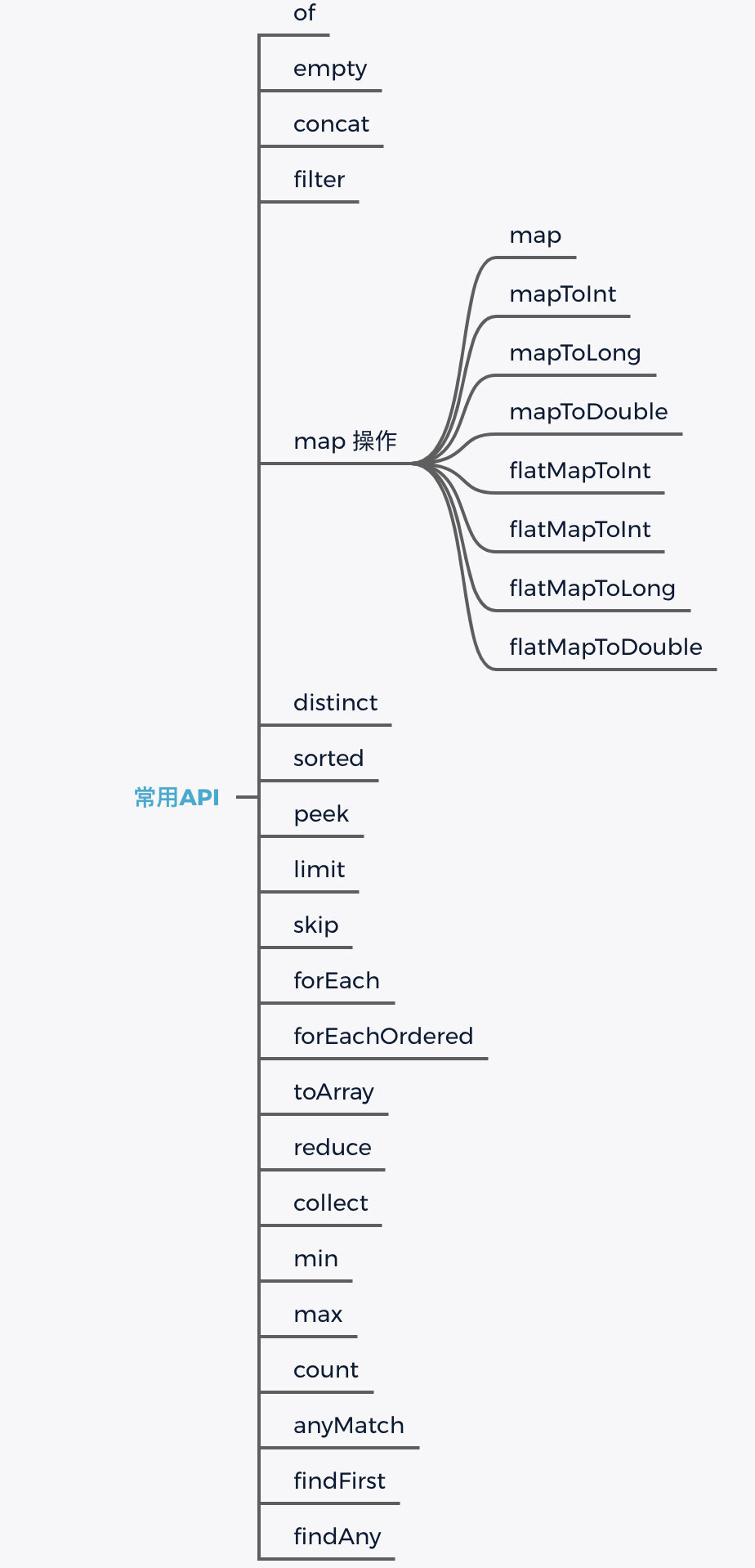### of

private static void createStream(){    Stream<String> stringStream = Stream.of("a","b","c");}

### concat

private static void concatStream(){    Stream<String> a = Stream.of("a","b","c");    Stream<String> b = Stream.of("d","e");    Stream<String> c = Stream.concat(a,b);}

### max

private static void max(){    Stream<Integer> integerStream = Stream.of(2, 2, 100, 5);    Integer max = integerStream.max(Integer::compareTo).get();    System.out.println(max);}

private static void max(){    Stream<Integer> integerStream = Stream.of(2, 2, 100, 5);    Comparator<Integer> comparator =  (x, y) -> (x.intValue() < y.intValue()) ? -1 : ((x.equals(y)) ? 0 : 1);    Integer max = integerStream.max(comparator).get();    System.out.println(max);}

### count

Stream<String> a = Stream.of("a", "b", "c");long x = a.count();

### peek

private static void peek() {    Stream<String> a = Stream.of("a", "b", "c");    List<String> list = a.peek(e->System.out.println(e.toUpperCase())).collect(Collectors.toList());}

### forEach

private static void forEach() {    Stream<String> a = Stream.of("a", "b", "c");    a.forEach(e->System.out.println(e.toUpperCase()));}

### forEachOrdered

Stream<String> a = Stream.of("a", "b", "c");a.parallel().forEach(e->System.out.println(e.toUpperCase()));

Stream<String> a = Stream.of("a", "b", "c");a.parallel().forEachOrdered(e->System.out.println(e.toUpperCase()));

### limit

private static void limit() {    Stream<String> a = Stream.of("a", "b", "c");    a.limit(2).forEach(e->System.out.println(e));}

### skip

private static void skip() {    Stream<String> a = Stream.of("a", "b", "c");    a.skip(2).forEach(e->System.out.println(e));}

### distinct

private static void distinct() {    Stream<String> a = Stream.of("a", "b", "c","b");    a.distinct().forEach(e->System.out.println(e));}

### sorted

private static void sorted() {    Stream<String> a = Stream.of("a", "c", "b");    a.sorted().forEach(e->System.out.println(e));}

private static void sortedWithComparator() {    Stream<String> a = Stream.of("a1", "c6", "b3");    a.sorted((x,y)->Integer.parseInt(x.substring(1))>Integer.parseInt(y.substring(1))?1:-1).forEach(e->System.out.println(e));}

private static List<User> getUserData() {    Random random = new Random();    List<User> users = new ArrayList<>();    for (int i = 1; i <= 10; i++) {        User user = new User();        user.setUserId(i);        user.setUserName(String.format("古时的风筝 %s 号", i));        user.setAge(random.nextInt(100));        user.setGender(i % 2);        user.setPhone("18812021111");        user.setAddress("无");        users.add(user);    }    return users;}

### filter

private static void filter(){    List<User> users = getUserData();    Stream<User> stream = users.stream();    stream.filter(user -> user.getGender().equals(0) && user.getAge()>50).forEach(e->System.out.println(e));    /**     *等同于下面这种形式 匿名内部类     *///    stream.filter(new Predicate<User>() {//        @Override//        public boolean test(User user) {//            return user.getGender().equals(0) && user.getAge()>50;//        }//    }).forEach(e->System.out.println(e));}

### map

map方法的接口方法声明如下，接受一个 Function函数式接口，把它翻译成映射最合适了，通过原始数据元素，映射出新的类型。

<R> Stream<R> map(Function<? super T, ? extends R> mapper);

Function的声明是这样的，观察 apply方法，接受一个 T 型参数，返回一个 R 型参数。用于将一个类型转换成另外一个类型正合适，这也是 map的初衷所在，用于改变当前元素的类型，例如将 Integer 转为 String类型，将 DAO 实体类型，转换为 DTO 实例类型。

@FunctionalInterfacepublic interface Function<T, R> {    /**     * Applies this function to the given argument.     *     * @param t the function argument     * @return the function result     */    R apply(T t);}

private static void map(){    List<User> users = getUserData();    Stream<User> stream = users.stream();    List<UserDto> userDtos = stream.map(user -> dao2Dto(user)).collect(Collectors.toList());}private static UserDto dao2Dto(User user){    UserDto dto = new UserDto();    BeanUtils.copyProperties(user, dto);    //其他额外处理    return dto;}

### flatMap

1. Stream<String[]>

2. Stream<Set<String>>

3. Stream<List<String>>

private static void flatMap(){    List<User> users = getUserData();    List<User> users1 = getUserData();    List<List<User>> userList = new ArrayList<>();    userList.add(users);    userList.add(users1);    Stream<List<User>> stream = userList.stream();    List<UserDto> userDtos = stream.flatMap(subUserList->subUserList.stream()).map(user -> dao2Dto(user)).collect(Collectors.toList());}

### collection

<R, A> R collect(Collector<? super T, A, R> collector);

private static void collect(){    Stream<Integer> integerStream = Stream.of(1,2,5,7,8,12,33);    List<Integer> list = integerStream.filter(s -> s.intValue()>7).collect(Collectors.toList());}

private static void collect(){    Stream<Integer> integerStream = Stream.of(1,2,5,7,8,12,33);    List<Integer> list = integerStream.filter(s -> s.intValue()>7).collect(ArrayList::new, ArrayList::add,            ArrayList::addAll);}

// 返回 userId:List<User>Map<String,List<User>> map = user.stream().collect(Collectors.groupingBy(User::getUserId));// 返回 userId:每组个数Map<String,Long> map = user.stream().collect(Collectors.groupingBy(User::getUserId,Collectors.counting()));

### toArray

collection是返回列表、map 等，toArray是返回数组，有两个重载，一个空参数，返回的是 Object[]

@FunctionalInterfacepublic interface IntFunction<R> {    /**     * Applies this function to the given argument.     *     * @param value the function argument     * @return the function result     */    R apply(int value);}

private static void toArray() {    List<User> users = getUserData();    Stream<User> stream = users.stream();    User[] userArray = stream.filter(user -> user.getGender().equals(0) && user.getAge() > 50).toArray(User[]::new);}

### reduce

private static void reduce(){    Stream<Integer> integerStream = Stream.of(1,2,5,7,8,12,33);    Integer sum = integerStream.reduce(0,(x,y)->x+y);    System.out.println(sum);}

## 并行 Stream

Stream 本质上来说就是用来做数据处理的，为了加快处理速度，Stream API 提供了并行处理 Stream 的方式。通过 users.parallelStream()或者users.stream().parallel() 的方式来创建并行 Stream 对象，支持的 API 和普通 Stream 几乎是一致的。

1. 必须在多核 CPU 下才使用并行 Stream，听上去好像是废话。

2. 在数据量不大的情况下使用普通串行 Stream 就可以了，使用并行 Stream 对性能影响不大。

3. CPU 密集型计算适合使用并行 Stream，而 IO 密集型使用并行 Stream 反而会更慢。

4. 虽然计算是并行的可能很快，但最后大多数时候还是要使用 collect合并的，如果合并代价很大，也不适合用并行 Stream。

5. 有些操作，比如 limit、 findFirst、forEachOrdered 等依赖于元素顺序的操作，都不适合用并行 Stream。

## 最后

Java 25 周岁了，有多少同学跟我一样在用 Java 8，还有多少同学再用更早的版本，请说出你的故事。## 评论 (2 条评论)「一个本打算写诗却写起了代码的田园码农」 代码也可以是“诗”呀哈哈13 分钟前

11 分钟前